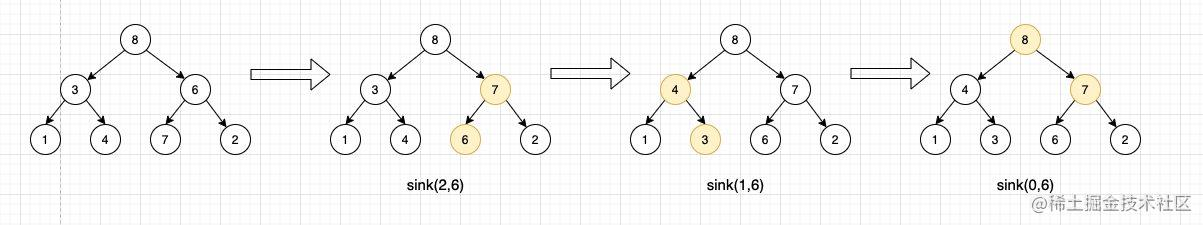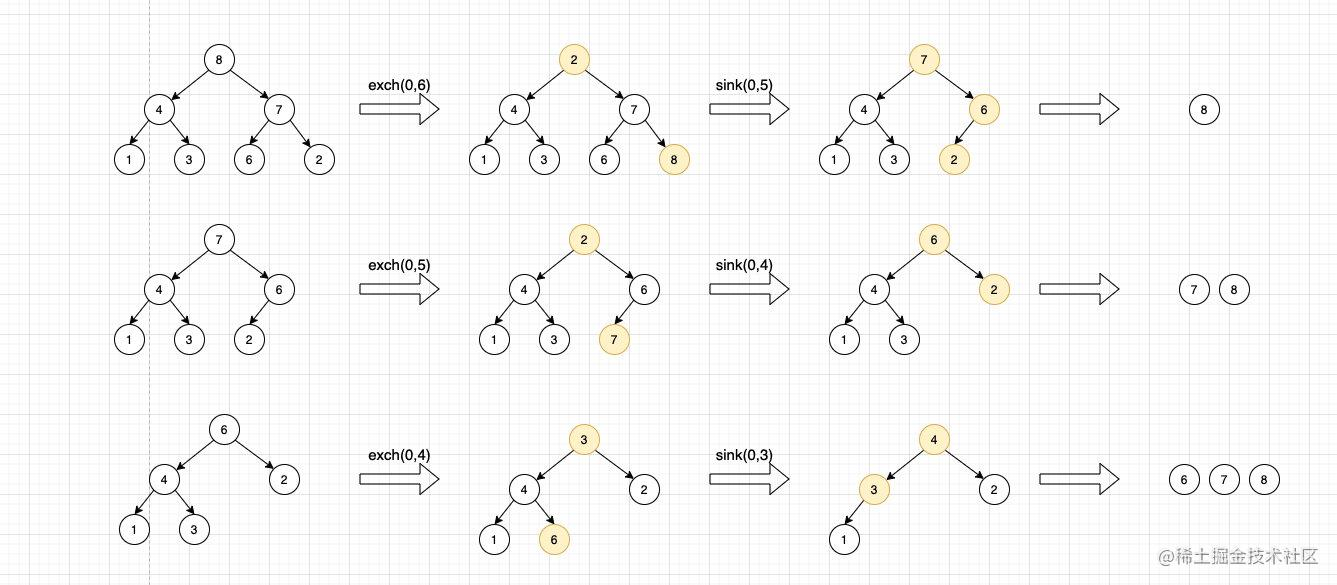# 图解堆排序## 前言

• 把原始数据构造成一个有序堆（构造堆）
• 从堆中按照递减顺序取出所有元素就可以得到排序结果（下沉排序）

``````private void sink(Comparable[] queue, int k, int size) {
while (2 * k + 1 < size) {
int i = 2 * k + 1;
if (i < size - 1 && less(queue[i], queue[i + 1])) {
i++;
}
if (less(queue[i], queue[k])) {
break;
}
exch(queue, i, k);
k = i;
}
}

## 构造堆

1. 从左向右遍历数组，然后把调用swim()上浮操作保证指针左边的元素都是堆有序的，就和优先级队列的插入过一样
2. 由于数组中的每个位置已经是堆的节点，我们可以从右向左调用sink()下沉操作构造堆，这个过程我们可以跳过子堆为1的元素，所以我们只需要扫描数组的一半元素。这种方式会更高效。## 下沉排序## 堆排序代码实现

``````@Override
public void sort(Comparable[] array) {
int size = array.length;
for (int k = size / 2; k >= 0; k--) {
sink(array, k, size);
}
while (size > 0) {
exch(array, 0, --size);
sink(array, 0, size);
}
}